# Sine Wave UPS

Today demand of pure sinewave inverter/UPS is increasing beacuse square wave UPS/Inverter can not run various equipments. So we have taken a sinewave UPS for testing.

We can know the purity of sine wave inveter by harmonics analysis. A square wave inverter has very high voltage THD at output whereas pure sinewave has zero THD(Ideal).

UPS Harmonic Test Report

Date : 11-11-2014

 Standard UL1778 article 49 Harmonic Distortion Test Instrument Under Test UPS 600VA Company Name Sukm Rated Voltage and Load 12V,50A Battery Type Tubular Battery Test Condition On battery source (Assuming full charge battery)

 Load Condition Voltage Voltage Harmonics 55.38 VA 227 V 2.843%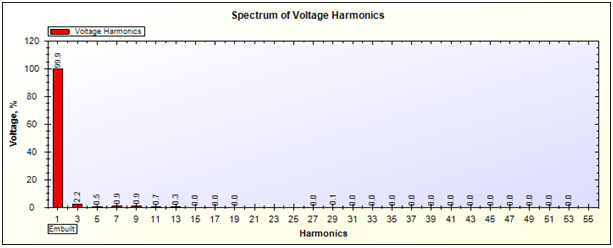1. Vthd is 2.843%.
2. Fundamental harmonics is 99.9 % of Vrms which is good.
3. Third harmonics is 2.2%
4. From 5th to 13th harmonics are  less than 1% of Vrms.
5. Other harmonics are zero.

 Load Condition(VA) Voltage Voltage Harmonics 124.78 227.3 2.611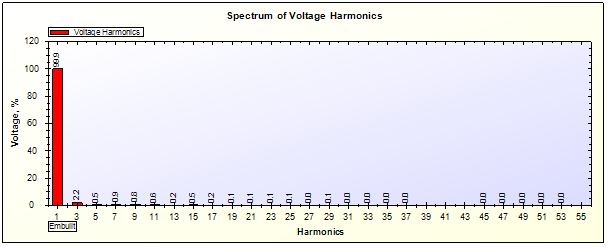Comment –

1. Vthd is 2.611%
2. First harmonics remain same on 99.9%.
3. Third harmonic is also same on 2.2%.
4.  15 to 25 harmonics are slightly increases.
5. Others are zero.

 Load Condition(VA) Voltage Voltage Harmonics 153.9 226.3 2.755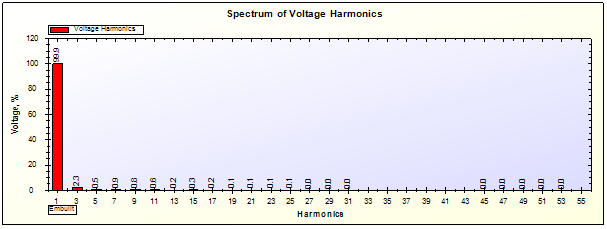Comment –

1. Vthd is 2.755%.
2. Fundamental harmonic is remain same.
3. Third harmonic increased by 0.1%.
4. Others are same as previous.

 Load Condition(VA) Voltage Voltage Harmonics 194.53 223.6 2.835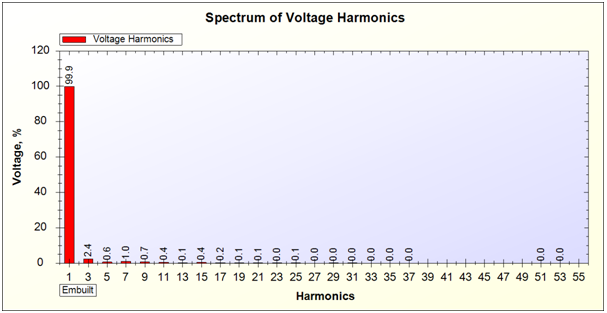Comment –

1. Vthd is 2.835%.
2. Third harmonic is again increased by 0.1%.
3. 5th to 17th harmonics are slightly increased.

 Load Condition(VA) Voltage Voltage Harmonics 302.8 222.8 3.596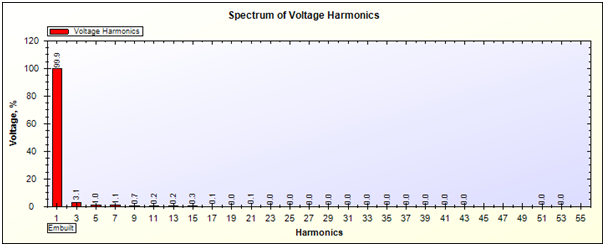Comment –

1. Vthd is 3.596%
2. Third harmonic is also increased by 0.7 %.
3. Harmonics from 5th to 15 are also increased.
4. Others are zero.

Summary –